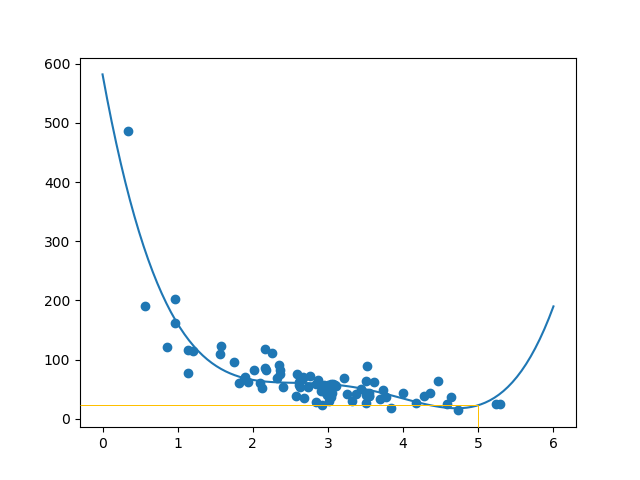# 机器学习 - 训练/测试

## 什么是训练/测试

80％ 用于训练，20％ 用于测试。

## 从数据集开始

### 实例

```import numpy
import matplotlib.pyplot as plt
numpy.random.seed(2)

x = numpy.random.normal(3, 1, 100)
y = numpy.random.normal(150, 40, 100) / x

plt.scatter(x, y)
plt.show()
```

### 结果：

x 轴表示购买前的分钟数。

y 轴表示在购买上花费的金额。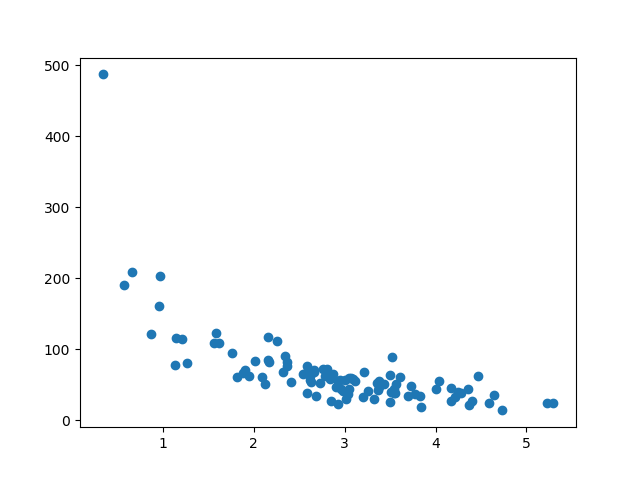## 拆分训练/测试

```train_x = x[:80]
train_y = y[:80]

test_x = x[80:]
test_y = y[80:]
```

## 显示训练集

### 实例

```plt.scatter(train_x, train_y)
plt.show()
```

### 结果：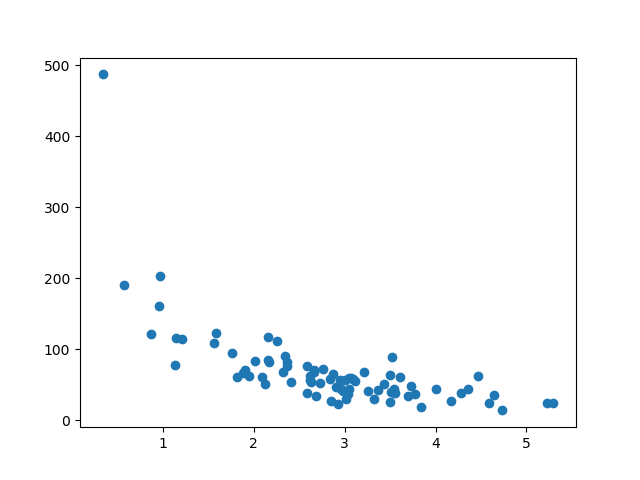## 显示测试集

### 实例

```plt.scatter(test_x, test_y)
plt.show()
```

### 结果：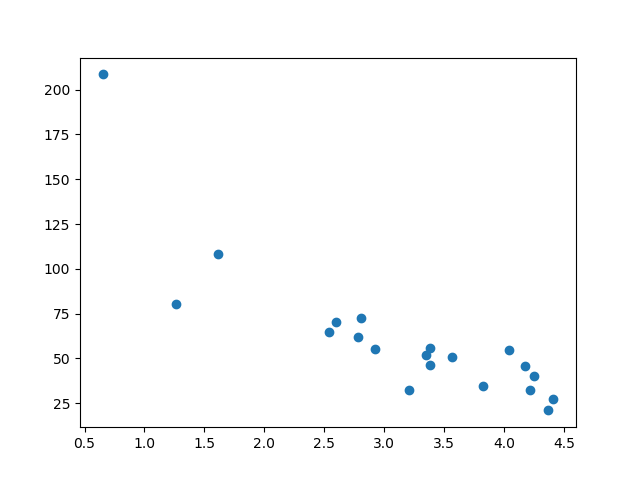## 拟合数据集

### 实例

```import numpy
import matplotlib.pyplot as plt
numpy.random.seed(2)

x = numpy.random.normal(3, 1, 100)
y = numpy.random.normal(150, 40, 100) / x

train_x = x[:80]
train_y = y[:80]

test_x = x[80:]
test_y = y[80:]

mymodel = numpy.poly1d(numpy.polyfit(train_x, train_y, 4))

myline = numpy.linspace(0, 6, 100)

plt.scatter(train_x, train_y)
plt.plot(myline, mymodel(myline))
plt.show()
```

### 结果：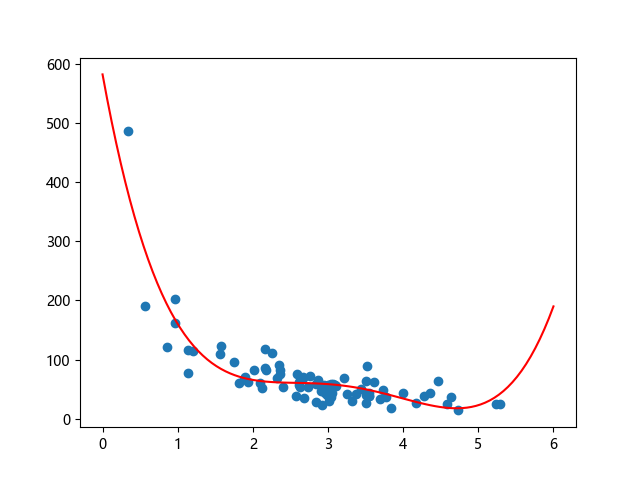## R2

sklearn 模块有一个名为 rs_score() 的方法，该方法将帮助我们找到这种关系。

### 实例

```import numpy
from sklearn.metrics import r2_score
numpy.random.seed(2)

x = numpy.random.normal(3, 1, 100)
y = numpy.random.normal(150, 40, 100) / x

train_x = x[:80]
train_y = y[:80]

test_x = x[80:]
test_y = y[80:]

mymodel = numpy.poly1d(numpy.polyfit(train_x, train_y, 4))

r2 = r2_score(train_y, mymodel(train_x))

print(r2)
```

## 引入测试集

### 实例

```import numpy
from sklearn.metrics import r2_score
numpy.random.seed(2)

x = numpy.random.normal(3, 1, 100)
y = numpy.random.normal(150, 40, 100) / x

train_x = x[:80]
train_y = y[:80]

test_x = x[80:]
test_y = y[80:]

mymodel = numpy.poly1d(numpy.polyfit(train_x, train_y, 4))

r2 = r2_score(test_y, mymodel(test_x))

print(r2)
```

## 预测值

### 实例

`print(mymodel(5))`